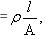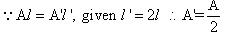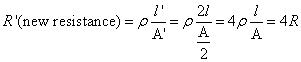Courses

# Test: Electricity And Circuits - 2

## 25 Questions MCQ Test NCERT Textbooks (Class 6 to Class 12) | Test: Electricity And Circuits - 2

Description
This mock test of Test: Electricity And Circuits - 2 for Class 6 helps you for every Class 6 entrance exam. This contains 25 Multiple Choice Questions for Class 6 Test: Electricity And Circuits - 2 (mcq) to study with solutions a complete question bank. The solved questions answers in this Test: Electricity And Circuits - 2 quiz give you a good mix of easy questions and tough questions. Class 6 students definitely take this Test: Electricity And Circuits - 2 exercise for a better result in the exam. You can find other Test: Electricity And Circuits - 2 extra questions, long questions & short questions for Class 6 on EduRev as well by searching above.
QUESTION: 1

### Resistance of a wire is r ohms. The wire is stretched to double its length, then its resistance in ohms is

Solution:

Resistancewhere d = length, and A= cross section area Now since the wire is stretched to double its length. the volume of the wire remains sameQUESTION: 2

### ----------- is the positive terminal of an electric cell.

Solution:

Metal cap is the positive terminal of the electric cell. The Metal disc is the negative terminal.

QUESTION: 3

### The tiny coiled metal wire present inside the bulb is called____________.

Solution:

An incandescent light bulb has a small thin wire with two bigger wires holding it up. This wire is called a filament. The filament is the part of the light bulb that produces light. Filaments in incandescent light bulbs are made of tungsten.

QUESTION: 4

A combination of two or more electric cells is called___________.

Solution:

A combination of two or more electric cells is called Battery

QUESTION: 5

An electric bulb has ____________terminals

Solution:
QUESTION: 6

----------- is an electric device which easily closes or opens an electric circuit.

Solution:
QUESTION: 7

A material which does not allow the electricity to pass through it is___________.

Solution:

An electrical insulator is a material whose internal electric charges do not flow freely; very little electric current will flow through it under the influence of an electric field.

QUESTION: 8

Non metals like __________and __________are conductors of electricity

Solution:

Carbon is the only gas, when solidified, that can conduct electricity. This is of the form Graphite, when it only has 3 bonds between the atoms. This leaves electrons to flow through the network structure.

QUESTION: 9

A material which allows the electricity to pass through it is ________.

Solution:

Conductors are the materials or substances which allow electricity to flow through them. They are able to conduct electricity because they allow electrons to flow inside them very easily.

QUESTION: 10

Electric energy is produced from the____________ within the cell.

Solution:

In simple cell battery converts chemical energy into electricity. The chemical reaction produces electrons, which then collect at the negative terminal of the battery. When there is a connection between the positive and negative terminal, the electrons flow to the positive terminal.

QUESTION: 11

A complete electric circuit is called ______________ electric circuit.

Solution:

Closed circuit means a complete electrical connection around which current flows or circulates.

QUESTION: 12

----------- is an insulator.

Solution:
QUESTION: 13

__________is a conductor.

Solution:
QUESTION: 14

The electric current in a closed circuit always flows from the ___________terminal of the electric cell to the ______terminal.

Solution:

The electric current always flows from the higher potential to lower potential. The positive terminal of an electric cell is at higher potential with respect to its negative terminal. Hence, in the given circuit, the current will flow from terminal P to terminal N.

QUESTION: 15

The base of an electric cell is its ___________terminal.

Solution:

Metal cap is the positive terminal and the metal disc is the negative terminal of the electric cell. An electric cell produces electricity from the chemicals stored inside it.

QUESTION: 16

The path along which electric current flows is_____________.

Solution:

Circuit A path through which electric charges flow. A continuous, unbroken path through which electrons can flow is a closed circuit. Charges, or current, can flow only through a closed circuit. A break or opening in a circuit creates an open circuit.

QUESTION: 17

In _____________ electrical energy is transformed in to mechanical energy.

Solution:

As the electrical energy is used to run blades in the mixer grinder and hence we say in mixer grinder electrical energy is transformed into mechanical energy(rotation of blades).

QUESTION: 18

------------- is an energy which flows in an electric circuit.

Solution:

An electric current is a flow of electric charge. In electric circuits this charge is often carried by moving electrons in a wire. It can also be carried by ions in an electrolyte, or by both ions and electrons such as in an ionised gas (plasma).

QUESTION: 19

The central _____________of an electric cell is always positive.

Solution:

Positive charge is up and the current is going to the negative charge. That's why the center terminal of an electric cell is always positive.

QUESTION: 20

Kerosene oil is an example of _______________.

Solution:

Petrol and kerosene oil have very low electrical conductivity and hence they are poor electrical conductors (i.e. insulators).

QUESTION: 21

The cylindrical container of electric cell is made of __________ and acts as negative terminal.

Solution:

Zinc due to electromagnetic series.

QUESTION: 22

The wire which carries away the current to the power house is called____________.

Solution:

The live wire carries current to the appliance at a high voltage. The neutral wire completes the circuit and carries current away from the appliance. The third wire, called the earth wire (green/yellow) is a safety wire and connects the metal case of the appliance to the earth.

QUESTION: 23

_____________ is a safety wire which protect us from electric shocks and electric fire.

Solution:

The main function of the earth wire is to conduct the leaking current from the electrical appliance to the ground . It is connected to the outer metallic body of the appliance . It is necessary to earth metallic appliances , as it protects the user from electric shocks by transferring the current to the earth.

QUESTION: 24

____________ is a device which converts chemical energy in to electrical energy.

Solution:

As electric cell contains chemicals in it which is present as chemical energy the cell converts it into electrical energy and the appliance uses the electrical energy because it cannot use the direct chemicals as there enegy

QUESTION: 25

An electric cell has __________ terminals.

Solution:

An electric cell has two terminals: Positive terminal and Negative terminal. Electricity to the bulb in a torch is provided by the electric cell. Electric cells are also used in alarm clocks, wristwatches, transistor radios, cameras and many other devices.